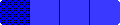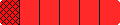Home

Grades 5-8 Fraction Bars Teacher's Guide

 Table of Contents Introduction  i-vii  (Eight lessons are linked below.)                                                                                                                Basic Concepts Step 1 Models for Fractions  1                                                  Step 2 Fractions for Regions and Sets  3 Step 3 Fraction Terminology and Notation  5 Step 4 Measuring with Fractions and Mixed Numbers  7 Step 5 Solving Problems Involving Regions and Sets  9 Equality Step 1 Models for Equality of Fractions  11 Step 2 Writing Equations for Equal Fractions  13 Step 3 Obtaining and Recognizing Equal Fractions  15 Step 4 Writing Fractions in Lowest Terms  17 Step 5 Finding Common Denominators for Fractions  19 Step 6 Solving Problems Involving Equality  21 Inequality Step 1 Models for Inequality of Fractions  23 Step 2 Determining Inequalities Using the ½ Benchmark  25 Step 3 Decreasing and Increasing Fractions  27 Step 4 Inequalities of Fractions and Mixed Numbers  29 Step 5 Rounding Fractions and Mixed Numbers  31 Step 6 Solving Problems Involving Inequality  33 Addition Step 1 Adding Fractions with Models  35 Step 2 Fractions and Mixed Numbers - Equal Denominators  37 Step 3 Adding Fractions - Unequal Denominators  39 Step 4 Adding Mixed Numbers - Unequal Denominators  41 Step 5 Solving Problems Involving Addition 43 Subtraction Step 1 Subtracting Fractions with Models 45 Step 2 Fractions and Mixed Numbers - Equal Denominators  47 Step 3 Subtracting Fractions - Unequal Denominators  49 Step 4 Subtracting Mixed Numbers - Unequal Denominators  51 Step 5 Solving Problems Involving Subtraction  53 Multiplication Step 1 Whole Numbers Times Fractions and Mixed Numbers  55 Step 2 Multiplying Fractions Times Whole Numbers  57 Step 3 Multiplying Fractions Times Fractions  59 Step 4 Mixed Numbers Times Mixed Numbers  61 Step 5 Solving Problems Involving Multiplication  63 Division Step 1 Dividing Fractions by Fractions  65 Step 2 Dividing by Fractions and Mixed Numbers  67 Step 3 Quotients of Whole Numbers that Equal Fractions  69 Step 4 Fractions as Ratios  71 Step 5 Solving Problems Involving Division  73 Fractions - Decimals - Percents Step 1 Connecting Fractions and Percents  75 Step 2 Connecting Fractions Decimals and Percents  77 Step 3 Number Lines with Decimals and Mixed Decimals  79 Step 4 Probability and Predictions  81 Step 5 Solving Problems Involving Probabilities as Fractions Decimals and Percents  83 Fraction Bars Games  85 - 111 Masters to Accompany Lessons  112 - 127    Followed by Answers for Masters

HomeHome